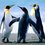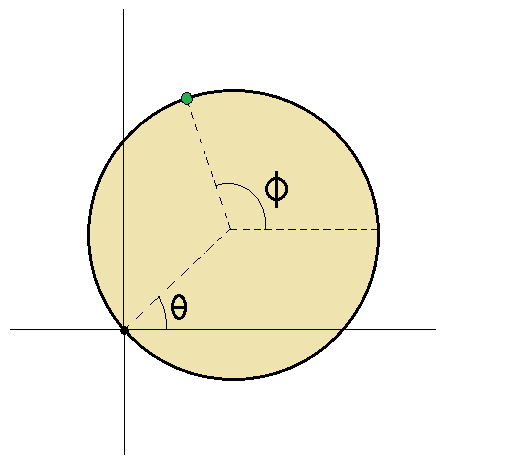# Mechanics problem

A horizontal circular disc of mass $M$ is free to rotate about a vertical axis through a point on its rim. If a dog(or pig if you wish) of mass $m$ walks once around the rim, by what angle does the rim turn through? Given that $\frac{M}{m}=\frac{8}{3}$.Note by Kudo Shinichi
2 years, 3 months ago

This discussion board is a place to discuss our Daily Challenges and the math and science related to those challenges. Explanations are more than just a solution — they should explain the steps and thinking strategies that you used to obtain the solution. Comments should further the discussion of math and science.

When posting on Brilliant:

• Use the emojis to react to an explanation, whether you're congratulating a job well done , or just really confused .
• Ask specific questions about the challenge or the steps in somebody's explanation. Well-posed questions can add a lot to the discussion, but posting "I don't understand!" doesn't help anyone.
• Try to contribute something new to the discussion, whether it is an extension, generalization or other idea related to the challenge.
• Stay on topic — we're all here to learn more about math and science, not to hear about your favorite get-rich-quick scheme or current world events.

MarkdownAppears as
*italics* or _italics_ italics
**bold** or __bold__ bold
- bulleted- list
• bulleted
• list
1. numbered2. list
1. numbered
2. list
Note: you must add a full line of space before and after lists for them to show up correctly
paragraph 1paragraph 2

paragraph 1

paragraph 2

[example link](https://brilliant.org)example link
> This is a quote
This is a quote
    # I indented these lines
# 4 spaces, and now they show
# up as a code block.

print "hello world"
# I indented these lines
# 4 spaces, and now they show
# up as a code block.

print "hello world"
MathAppears as
Remember to wrap math in $$ ... $$ or $ ... $ to ensure proper formatting.
2 \times 3 $2 \times 3$
2^{34} $2^{34}$
a_{i-1} $a_{i-1}$
\frac{2}{3} $\frac{2}{3}$
\sqrt{2} $\sqrt{2}$
\sum_{i=1}^3 $\sum_{i=1}^3$
\sin \theta $\sin \theta$
\boxed{123} $\boxed{123}$

## Comments

Sort by:

Top Newest

A uniform circular disk of mass $M$ and radius $R$ is confined to the $xy$ plane. It rotates with no frictional losses about the $z$ axis through a point on its edge. Gravity is into the page, and is thus irrelevant to this situation.

A bead of mass $m$ slides along a friction-less track on the circumference of the disk. The angle $\theta$ is the angle between the positive $x$ axis and a line from the origin to the center of the disk. The angle $\phi$ is the angle between the positive $x$ axis and a line from the the center of the disk to the bead.Coordinates and velocities of the bead:

$x = R \, cos \theta + R \, cos \phi \\ y = R \, sin \theta + R \, sin \phi \\ \dot{x} = -R \, sin \theta \, \dot{\theta} - R \, sin \phi \, \dot{\phi} \\ \dot{y} = R \, cos \theta \, \dot{\theta} + R \, cos \phi \, \dot{\phi}$

Kinetic Energy of the system:

$E = \frac{1}{2} m (\dot{x}^2 + \dot{y}^2) + \frac{1}{2} \Big(\frac{1}{2} M R^2 + M R^2 \Big ) \dot{\theta}^2 \\ = \Big (\frac{1}{2} m R^2 + \frac{3}{4} M R^2 \Big) \dot{\theta}^2 + \frac{1}{2} m R^2 \dot{\phi}^2 + m R^2 \, sin\theta \, sin\phi \, \dot{\theta} \, \dot{\phi} + m R^2 \, cos\theta \, cos\phi \, \dot{\theta} \, \dot{\phi}$

Since all of the mass is at the same height with respect to gravity, the system Lagrangian is equal to the kinetic energy.

$L = E$

Equations of motion:

$\frac{d}{dt} \frac{\partial{L}}{\partial{\dot{\theta}}} = \frac{\partial{L}}{\partial{\theta}} \\ \frac{d}{dt} \frac{\partial{L}}{\partial{\dot{\phi}}} = \frac{\partial{L}}{\partial{\phi}}$

Evaluating the equations of motion yields the dynamics:

$\Big(m + \frac{3}{2} M \Big) \, \ddot{\theta} + m(sin\theta \, sin \phi + cos\theta \, cos\phi) \, \ddot{\phi} = m (cos\theta \, sin\phi - sin\theta \, cos\phi) \dot{\phi}^2 \\ m(sin\theta \, sin \phi + cos\theta \, cos\phi) \, \ddot{\theta} + m \ddot{\phi} = m (sin\theta \, cos\phi - cos\theta \, sin\phi) \dot{\theta}^2$

Initial conditions:

$\theta_0 = 0 \\ \dot{\theta}_0 = 0 \\ \phi_0 = 0 \\ \dot{\phi}_0 \neq 0$

We are interested in the angle $\theta$ which has elapsed by the time $\phi - \theta = 2 \pi$, meaning that the bead has made a full revolution around the disk circumference. For $M = \frac{8}{3} m$, the simulation is not very well behaved for some reason (it doesn't converge very well as the time step is varied). I will post a problem later asking for the results for the $M = 5 m$ case, since this case is very well behaved.

- 2 years, 3 months ago

Log in to reply

Yeah u r right sir , thx a lot.

- 2 years, 3 months ago

Log in to reply

Any ideas to solve this problem?? @Mark Hennings Sir @Steven Chase sir pls share your ideas @Aaghaz Mahajan pls help ?

- 2 years, 3 months ago

Log in to reply

Let's say we have a smooth track on the edge of the disk that a bead slides on. Do you regard that as being an equivalent problem?

- 2 years, 3 months ago

Log in to reply

Yeah exactly same @Steven Chase sir.

- 2 years, 3 months ago

Log in to reply

@Steven Chase sir u got it ?

- 2 years, 3 months ago

Log in to reply

Yes, I'm going to do one more derivation to make sure I have it right. Then I'll post it. A bit of warning in advance; it doesn't boil down to a nice simple expression.

- 2 years, 3 months ago

Log in to reply

I didn't know the answer , likely some other info must be given to cause it to become easy .

- 2 years, 3 months ago

Log in to reply

×

Problem Loading...

Note Loading...

Set Loading...